# Experimental Investigations of Al-Cr3C2 Composite Preform Densification and Deformation

Experimental Investigations of Al-Cr3C2 Composite Preform Densification and Deformation

Department of Mechanical Engineering, Acharya Nagarjuna University, Nagarjunanagar 522 510, Guntur, A.P., India

Department of Mechanical Engineering, R.V.R. & J.C. College of Engineering, Guntur 522019, A.P., India

Corresponding Author Email:
Page:
185-192
|
DOI:
https://doi.org/10.18280/acsm.460403
4 May 2022
|
Accepted:
23 July 2022
|
Published:
31 August 2022
| Citation

OPEN ACCESS

Abstract:

This investigation on strain hardening, densification and workability of the Sintered aluminium- Chromium Carbide composition of (Al-Cr3C2 of 2, 4 and 6%) preforms subjected to upsetting were investigated in this research. Industrial practitioners needed the workability data and densification mechanisms to plan and envisage the failure strains. In the current study, under triaxial stress state conditions Al-Cr3C2 preform with primary preform densities and various aspect ratios were compressed. Strain hardening, densification behaviors of aluminium- Chromium Carbide be investigated by gradually increasing the load till the fracture occurs. The outcome of adding Cr3C2 to Al and the impact of aspect ratio on formability was also extensively investigated. We looked at the parameters of stress ratio, instant varying strain rate, work hardening exponent, instantaneous density coefficient and densification attained.

Keywords:

powder metallurgy, Aluminium-Cr3C2 preforms, cold upsetting, instantaneous strain rate sensitivity & strain hardening index

1. Introduction

Aluminum is useful for a wide range of applications because of its light weight, ductility, durability, malleability, and nonmagnetic characteristics. The low density and corrosion resistance of aluminium are well-known. Because aluminium is one-third the density and rigidity of steel, it can be used in a variety of applications. Aluminum and its alloys are machinable, castable, drawable, and extrudable. Aluminum metal was used in alloying and composite preparation to increase material qualities for a variety of applications . Sumathi and Selvakumar  investigated the deformation characteristics by using cold upsetting process for sintered Cu-Sic. The conclusions of the study are at lower values of Sic the deformation is high at constant initial fractional density. Doraivelu et al.  presented a yield theory based solely on the uniaxial compressive stress condition. workability factor (β) which indicates workability factor suggested for the study of upsetting of compacts formability, Impact of stress ratio parameters, geometrical shape factor and barreling effects of densification of Al-Al2O3  was investigated by Narayanasamy et al. . Components made with powder metallurgy (PM) have better properties than those made with traditional manufacturing methods. Powder metallurgy production is quick and cost – effective, making it ideal for high – volume manufacturing with minimal powder contamination were investigated by Inigoraj et al. . Kaku et al.  analyzed temperature changes of densification behaviour on aluminium metal matrix composites using powder metallurgical route. The influence of mechanical working on deformed preforms is found using Potentio-dynamic polarization method. The conclusion of the study is there is reduction in corrosion rate with increase of deformation. Narayanasamy et al.  suggested a mathematical theory of plasticity on compressed materials used for PM. Sljapic et al.  studied fracture emergence during axisymmetric and non-axisymmetric cold forming of brass. Bao  discovered a link between stress and corresponding strain for production of cracks. Narayan and Rajeshkannan  analyzed carbon effects on the behaviour of cylindrical preforms made of cold upsetting and sintering. For the study the different carbon contents of 0%, 0.35%, 0.75% and 1.1% with 0.4 aspect ratio and theoretical density of 84% is considered. Gouveia et al.  investigated various materials of numerous geometries to determine the fracture damage of various stacking conditions. Because of closure of pores, the volume of the samples was reduced. As a result, it was discovered that the creation of P/M preforms deviates from volume constancy rules, and that material properties vary in tandem with porosity changes. Equivalent strain and stress triaxiality have also been discovered to be important factors. According to the scientists, the characteristics ratio of stress and strain were the primary cause of secondary consequences are investigated by Narayanasamy et al. . They also investigated the formability behaviour which varied according to aspect ratio and size of iron particles using Upsetting tests. Narayanasamy et al. [14-17] who discovered that the aspect ratio, geometry, size of the particle, reinforcement percentage, geometry of the die, the type of lubricants used, and pressing weight effects workability of various P/M components. According to Taha et al. , lowering particle size and volume percentage has a beneficial effect on workability index. Bensam Raj et al.  studied the variations in various parameters and the effective time intervals and sintering temperature on study of Al – SiC composites manufactured by cold upsetting operation in PM. For the study three levels of sintering temperatures and varying SiC reinforcement’s content (0%, 10%, 20%) are considered. Ramadurai et al.  investigated the wear behaviour in iron metal matrix composites by taking iron as matrix and alumina and bagasse fly ash as all elements using the powder metallurgy. The SEM analysis is carried out to identify the distribution of elements. Selvam and Singh  studied the densification behaviour of sintered zinc and zinc oxide composites based on initially preformed density and initial aspect ratio. The results show that the composites show better performance than zinc dust. the higher stress and strain values are obtained at 150℃ in comparison with 200℃ and 250℃. The aspect ratios are varied between 0.4 – 0.85. Venkatesh et al.  manufactured the Al – ZrB2 composites powder metallurgical route using different proportions of powdered ZrB2, to study the aspect ratios 0.35, 0.5 and 0.65 are considered. As per the results formability stress is directly proportional to preform fractional density and inversely proportional to aspect ratio also very higher fracture strain obtained for preforms of min aspect ratios and high density. The growth in ZrB2 reinforcement effects for raise in hardness of composite. Lou et al.  analyzed lubrication effects of densification behaviour of powdered Titanium and micro structural changes due to sintering. The samples are made by cold compact of titanium powder and 0-2 wt% stearic acid or magnesium stearate. The results reveal that the compressibility is improved at 0.3-0.6 wt% and also there is more uniform density distribution of compact. Selvakumar et al.  analyzed workability & strain hardening of Fe-C- Mn sintered powder preforms using cold deformation process. The results show that the performance of Fe - 0.10%C - 0.70% Mn having maximum initial preform density is better greater formability values. Ramesh et al.  investigated behaviour of Aluminium and Al – 5% SiC made using powder metallurgy route by cold compacting. The densification during compact is measured by using mass constancy principle. Ravichandran et al.  examine the workability behaviour of Al- 2.5% TiO2 - Gr composites using techniques of PM. From the results, observed that mixing of alloying elements improves stress ratio parameters significantly. Powder metallurgy  was employed to investigate the densification behaviour of sintered low alloy steels with Ni and Cr during cold and hot deformation according to the findings. The inclusion of alloying elements has a substantial impact on the deformation behaviour of alloy preforms. Current study looks at the workability of Al+2%Cr3C2, Al+4%Cr3C2, Al+6%Cr3C2 composite preforms with altered aspect ratios. Various stresses, strains, densities reached by the preforms have been measured during upsetting studies.

Shahid et al.  investigated the porosity, density, wear and hardness of aluminium matrix hybrid composites with addition of varying amounts of graphite. The conclusions of the study are the composition with 5% volume of graphite showed better performance in terms of improved mechanical properties.

2. Experimental Work

99.7% Pure atomized aluminium powder which is 46 μm of particle size considered as the matrix material and rutile phase of Cr3C2 powder taken as reinforcement material. Chromium carbide and aluminium powder were both oxidized to some extent. The desired amount of Al-Cr3C2 powders were weighed and manually stirred in a porcelain bowl. The Al-Cr3C2 (of 2, 4, 6%), of weight were poured into a die of 16 mm diameter and 70 mm length and the coatings of zinc stearate are applied on die walls. Different aspect ratios were used to create the preforms of 0.5, 0.75 and 1 on a 600 KN universal testing machine under 30 KN pressure to the required density. Table 1 shows a schematic representation of powder compacts as well as initial conditions. Under a controlled atmosphere in a tubular furnace, the compacts were sintered at 530℃ for 90 minutes. The sintered preforms were incrementally presses in a universal testing machine to various height reductions. Increased loads were applied to each billet till free surface is exposed to crack. For each deformed compacts, (Hf) final height, (Dtop) top contact diameter, (Dbottom) bottom contact diameter, (Db) bulge diameter were considered using a vernier calipers, and the density was measured using Archimedes’ principle at every stage during deformation. The (σz) normal stress, (εz) normal strain, (σm) hydrostatic stress, (σθ) hoop stress, (εθ) hoop strain, (σeff) effective stress, (εeff) effective strain, (ni) instantaneous strain hardening and (Ai) instantaneous density coefficient were all calculated using the measured dimensions.

Table 1. Initial conditions for Al-Cr3C2 powder compacts

## t1.jpgAspect Ratio ho-Height (mm) Do-Diameter (mm) Preform initial density Al-4%Cr3C2 1 15.7 16 0.8 0.75 11.9 16 0.8 0.5 7.8 16 0.8 Al-6%Cr3C2 1 15.8 16 0.8 0.75 12 16 0.8 0.5 8 16 0.8
3. Theoretical Analysis

Narayanasamy et al.  provided the mathematical equations for determining different upsetting parameters in a triaxial stress state.

True axial strain (εz) is given as:

$\varepsilon_z=\ln \left(\frac{H_f}{H_0}\right)$          (1)

where, Ho -Preforms' initial height; Hf  - Preforms' final height.

The Hoop Strain $\left(\varepsilon_\theta\right)=\ln \left[\frac{2 D_b^2+D_c^2}{3 D_0^2}\right]$          (2)

where, Do - Preforms' initial diameter; Db - Preforms' bulge diameter; DC - Preforms' contact diameter.

For triaxial stress state condition, the final diameter and related hoop strain increases during upsetting .

$\alpha=\left[\frac{\mathrm{A}}{\mathrm{B}}\right]$         (3)

$A=\left[\left(2+R^2\right) \sigma_\theta-R^2\left(\sigma_z+2 \sigma_\theta\right)\right]$          (4)

B=[(2+R2)σzR2(σz+2σθ)]          (5)

where, α -Poisson’s ratio; R - Relative density; σz - Axial stress; σθ - Hoop stress.

$\sigma_\theta=\left(\frac{2 \alpha+\mathrm{R}^2}{2-\mathrm{R}^2+2 \alpha \mathrm{R}^2}\right) \sigma_{\mathrm{z}}$          (6)

$\sigma_m=\frac{\left(\sigma_Z+2 \sigma_\theta\right)}{3}$          (7)

$\left(\sigma_{e f f}\right)=\sqrt{\left[\frac{\sigma_{\mathrm{z}}^2+2 \sigma 2{ }_\theta-\mathrm{R}^2\left(\sigma_\theta^2+2 \sigma_{\mathrm{Z}} \sigma_\theta)\right.}{\left(2 \mathrm{R}^2-1\right)}\right]}$           (8)

$\beta=\left(\frac{3 \sigma_m}{\sigma_{e f f}}\right)$          (9)

$\varepsilon_{e f f}=\left(\frac{2}{3\left(2+R^2\right)}\left[2 \varepsilon_\theta^2+2 \varepsilon_{\mathrm{z}}^2-4 \varepsilon_\theta \varepsilon_{\mathrm{z}}\right]+\frac{\left(\varepsilon_{\mathrm{z}}+2 \varepsilon_\theta\right)^2}{3}\left(1-R^2\right)\right)^{1 / 2}$          (10)

έeff=$\left(\frac{2}{3\left(2+R^2\right)}\left[2 \stackrel{'}{\varepsilon}_\theta^2+2 \stackrel{'}{\varepsilon}_{\mathrm{z}}^2-4 \stackrel{'}{\varepsilon}_\theta \stackrel{'}{\varepsilon}_{\mathrm{z}}\right]+\frac{\left(\stackrel{'}{\varepsilon}_{\mathrm{z}}+2 \stackrel{'}{\varepsilon}_\theta\right)^2}{3}\left(1-R^2\right)\right)^{1 / 2}$          (11)

3.1 Constitutive relationship

For sintered preforms, the constitutive relationship as per the findings of Narayanasamy et al. .

$\sigma_{e f f}=\mathrm{KR}^{\mathrm{A}} \varepsilon_{e f f}^n \stackrel{'}{\varepsilon}_{e f f}^m$          (12)

where, σeff - Effective stress; Ki - Instantaneous Strength Coefficient; R - Relative density; εeff - Effective strain; έeff - Effective strain rate; Ai - Instantaneous Density Coefficient; ni - Instantaneous Strain hardening exponent; mi - Instantaneous Strain rate sensitivity.

$A_i=\frac{\ln \left(\frac{\sigma_i}{\sigma_i-1}\right)}{\ln \left(\frac{R_i}{R_i-1}\right)}$          (13)

$n_i=\frac{\ln \left(\frac{\sigma_i}{\sigma_i-1}\right)}{\ln \left(\frac{\varepsilon_i}{\varepsilon_i-1}\right)}$          (14)

$m_i=\frac{\ln \left(\frac{\sigma_i}{\sigma_i-1}\right)}{\ln \left(\frac{\stackrel{'}{\varepsilon}_i}{\stackrel{'}\varepsilon_i-1}\right)}$          (15)

$K_i=\left(\frac{\sigma}{R^2 \varepsilon^n \stackrel{'}{\varepsilon}^m}\right)$          (16)

Ai, ni, Ki and mi are some of the deformation parameters (constants) that can be calculated from the above equations.

4. Results and Discussions

Figure 1 represents Sem image Al–Cr3C2 composite. Figure 2(a), (b) represents the Pre and post de-formation of an upset preform. For compacts of Al+2%Cr3C2, Al+4%Cr3C2, Al+6%Cr3C2 composition with an aspect ratio of 0.5, Figure 3 shows relationship between the axial, hydrostatic, hoop and effective stresses and axial strains. Because the hydrostatic stress is greater than the effective and hoop stresses, the axial stress is compressive. With an increase in axial strain, all stresses have increased. It's also worth noting that the strain-to-failure ratio has diminished as Cr3C2 level has increased. This is owing to the Cr3C2 powder's ability to reinforce the aluminium matrix. It also shows how well the Cr3C2 has blended into the matrix. According to the experiments, the preforms with lower aspect ratios is greater load-bearing capability when compared to that of the preforms of high aspect ratios for the compositions Aluminium+2% Cr3C2, Aluminium+4% Cr3C2 and Aluminium+6% Cr3C2 with varying aspect ratios of 0.5, 0.75, 1.0. Figure 4a, 4b and 4c have been shown the relationship between the formability stress index (β) vs relative density (R). It's worth noting that the (β) and R are directly proportional to each other.

## 1.jpg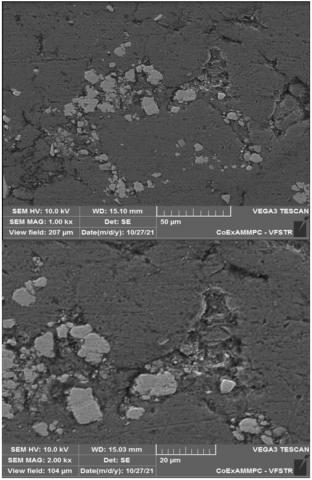Figure 1. Sem image Al–Cr3C2 composite

## 2.jpg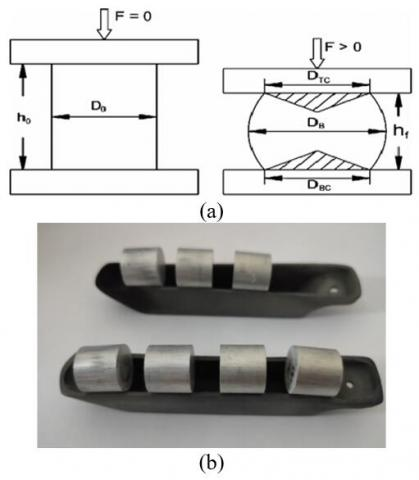Figure 2. Pre and post de-formation of an upset preform

## 3.jpg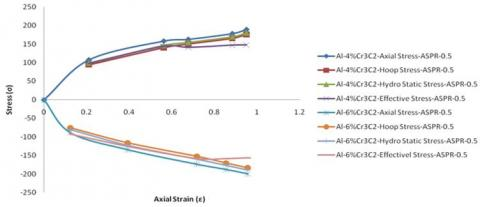Figure 3. Plot of Aluminium - 4%, 6% Cr3C2 for a 0.5 aspect ratio, various stresses and axial strain

## 4a.jpg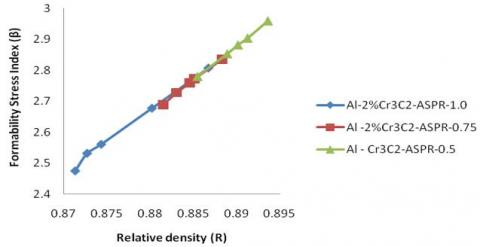(a)

## 4b.jpg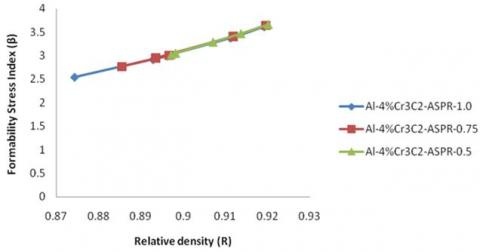(b)

## 4c.jpg(c)

Figure 4. a, b, c plot of the (R) and (β) values of (Al-2%, 4%, 6%Cr3C2) for various aspect ratios such as 1.0, 0.75 and 0.5

The formability index of Cu-Tic preforms was predicted using statistical methods by Narayanasamy et al. . In this project, too, a similar approach was conducted. Curve fitting techniques such as, exponential, polynomial and power law relationships were used. Polynomial fitting is the best method for predicting results that are nearer to the real since the correlation coefficient R2 value is approaching 1, as per the data of Table 2. Lower aspect ratio preforms densify faster than higher aspect ratio preforms. The authors  noticed that by pushing the metal in the center towards the edge, the deformation of the metal starts from center to the outer perimeter of the preforms. Forming load is available to the center of a low aspect ratio preform sooner than it is to its higher equivalent. According to these conditions, there will be disruption of pores by forming forces, compress the preform in axial direction, and pushes it to the outside perimeter. As a result, a preform with a low aspect ratio densifies more when compared to higher values. Furthermore, it was discovered that increasing the Cr3C2 content reduced the final density.

Figures 5a, 5b & 5c have been shown the relationship between the (R) and the (Ai) for Aluminium + 2% Cr3C2, Aluminium + 4% Cr3C2 and Aluminium + 6% Cr3C2 with varied aspect ratios of 0.5, 0.75, and 1.0. With an increment in relative density, Ai increases somewhat before rapidly decreasing, as shown in Figure 5a, preforms made of Aluminum + 2% Cr3C2 with an aspect ratio of 0.5 are almost identical. The instantaneous density coefficient rises and then falls for samples with ASPR of 1.0 and 0.75 for the same composition. The instantaneous density coefficient value increases slowly before rapidly falling with an ASPR of 1.0.as seen in Figure 5b. Preforms with different aspect ratios follow the same pattern. The (Ai) is an amount of the preforms' densification as a result of incremental forming stress. In early stages of the upsetting process this number is large because the forming load is used to seal the pores. The ensuring forming load squeezes the metal in a radial manner once the pores are closed, enhancing densification. Low aspect ratio preforms are of lowest (Ai) value, which depicts high densification despite the presence of Cr3C2. For an aspect ratio of 1.0, the Ai significance develops gradually before quickly decreasing as shown in Figure 5c.

## 5a.jpg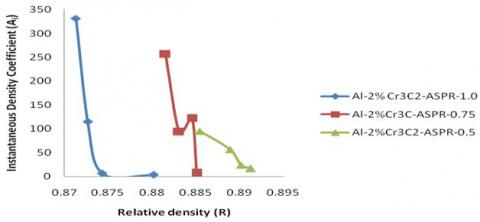(a)

## 5b.jpg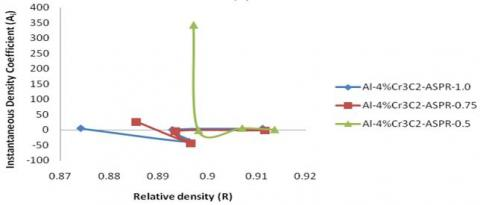(b)

## 5c.jpg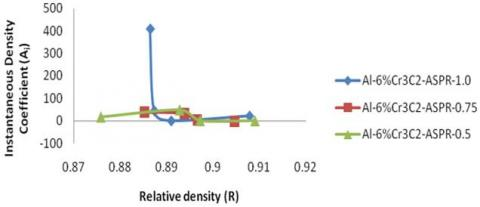(c)

Figure 5. a, b, c graph for (R) vs (Ai) values of (Al-2%, 4%, 6%Cr3C2) for various AR such as 1.0, 0.75 and 0.5

In preforms with different aspect ratios, a similar pattern can be noticed. The instantaneous density coefficient (Ai) is a measurement of preform densification caused by incremental forming stress. Because the forming load is utilised to seal the pores during the initial phases of the upsetting process, this figure is high. The forming load squeezes the metal in a radial direction once the pores are closed, resulting in greater densification. Despite the presence of Cr3C2, the lowest Ai value is found in preforms with low aspect ratios, indicating that densification should be improved.

The association between (ni) and (R) relative density has been plotted in Figures 6a, 6b & 6c for various aspect ratios 1.0, 0.75 and 0.5 of Al -Cr3C2 (2, 4 & 6%) preforms, respectively. The (ni) drops fast as the (R) increases. The (ni) index value for Aluminium – 6% Cr3C2 preforms drops for aspect ratios of 0.75 and is constant for further ASPR. The (ni) value lowers at the conclusion of the deformation, when fracture initiation begins.

## 6a.jpg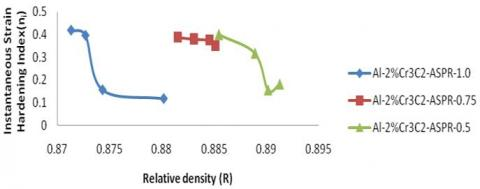(a)

## 6b.jpg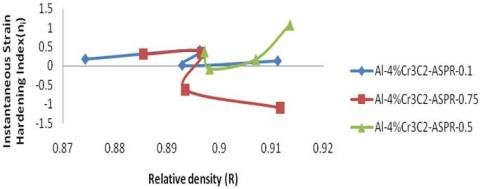(b)

## 6c.jpg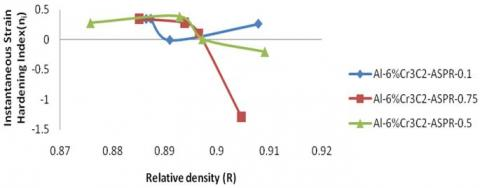(c)

Figure 6. a, b, c graph of (R) vs (ni) values for (Al-2%, 4%, 6%Cr3C2) of various AR such as 1.0, 0.75 and 0.5

## 7a.jpg(a)

## 7b.jpg(b)

## 7c.jpg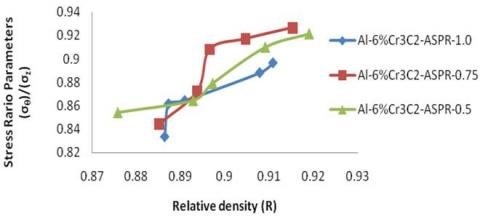(c)

Figure 7. a, b, c graph (R) vs (σθ)/(σz) of (Al-2%, 4%, 6%Cr3C2) for various AR- 1.0, 0.75 and 0.5

## 8a.jpg(a)

## 8b.jpg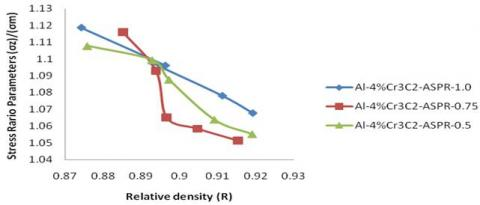(b)

## 8c.jpg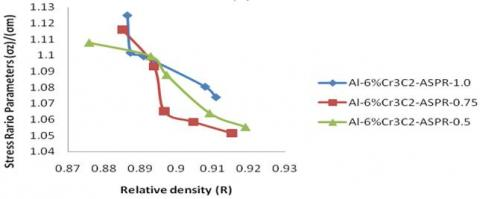(c)

Figure 8. a, b, c graph (R) vs (σz)/(σm) of (Al-2%, 4%, 6%Cr3C2) for various AR

## 9a.jpg(a)

## 9b.jpg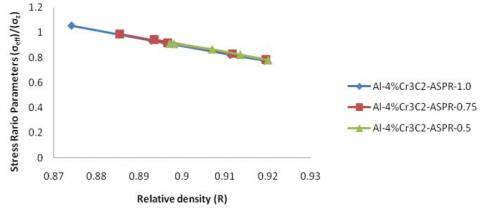(b)

## 9c.jpg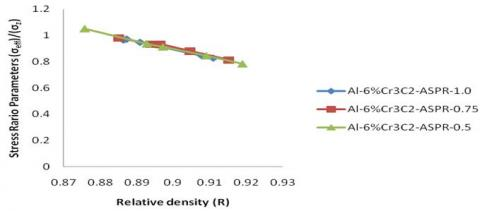(c)

Figure 9. a, b, c graph (R) vs (σeff)/(σz) of (Al-2%, 4%, 6%Cr3C2) for various AR - 1.0, 0.75 and 0.5

Figures 7a, 7b & 7c have been shown the relationship between the ratios of stress parameters (σθ/σz) vs relative density (R) for Aluminium + 2% Cr3C2, Aluminium + 4% Cr3C2, Aluminium + 6% Cr3C2, aspect ratios in preforms 1.0, 0.75 & 1.0 respectively. For each aspect ratio, the stress ratio parameter rises as relative density rises. This is because aluminium contains Cr3C2 particles, which affect flow behaviour.

Figure 8a, 8b and 8c show the (σz/σm) vs (R) for Aluminium + 2% Cr3C2, Aluminium + 4% Cr3C2, Aluminium + 6% Cr3C2 aspect ratio preforms of 1.0, 0.75, and 0.5, respectively. Figures show that as relative density increases, (σz/σm) decrements rapidly. As per the graphs, adding Cr3C2 to aluminum completely changes the densification process. The presence of Cr3C2 particles interferes with the flow of aluminum.

Figures 10a, 10b, and 10c show the (σeff/σz) and (R) of Al - Cr3C2 of 2, 4 & 6% preforms with different ASPR of 1.0, 0.75, and 0.5. The graphs illustrate that the stress ratio parameter rapidly falls as the relative density increases. When Cr3C2 particles are added to aluminium, the densification process is altered, resulting in a wide range of plasticity. Furthermore, when Cr3C2 particles are present in the aluminium, the stress ratio metric (σeff/σz) is lowered.

## 10.jpg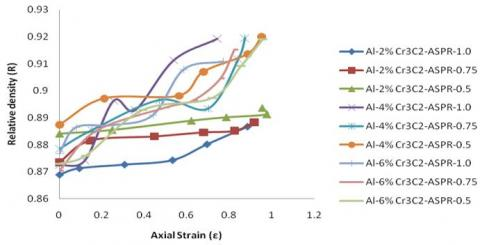Figure 10. Plot of the axial strain (ε) and (R)

Table 2. Different aspect ratios and compositions, the best fit curves for the correlation between the value of the (β) and the (R) (Figure 4 a, b, c)

 Composition Aspect Ratio Curve Fitting Curve Equation R2 Al–2% Cr3C2 1 Polynomial y=233.2x2-430.7x+195.7 R²=0.995 0.75 Polynomial y=464.9x2-844.3x+380.3 R²=0.999 0.5 Polynomial y=139.5x2-226.4x+93.86 R²=0.999 Al–4% Cr3C2 1 Polynomial y=145.7x2-237.6x+98.87 R²=0.999 0.75 Polynomial y=161.6x2-266.1x+111.7 R²=0.999 0.5 Polynomial y=95.72x2-146.1x+57.07 R²=0.999 Al–6% Cr3C2 1 Polynomial y=59.26x2-82.11x+28.99 R²=0.999 0.75 Polynomial y=84.01x2-125.7x+48.19 R²=0.999 0.5 Polynomial y=160.9x2-264.6x+110.9 R²=1

Table 3. Different aspect ratios and compositions, the best fit curves for the relationship between the value of the relationship of (σθ/σz) and (R) (Figure 7 a, b, c)

 Composition Aspect Ratio Curve Fitting Curve Equation R2 Al – 2% Cr3C2 1 Polynomial y=492.0x2-868.4x+382.3 R²=0.786 0.75 Polynomial y=930.1x2-1649.x+730.1 R²=0.972 0.5 Polynomial y=44.45x2-77.01x+34.23 R²=0.907 Al – 4% Cr3C2 1 Polynomial y=4.837x2-7.270x+3.499 R²=0.998 0.75 Polynomial y=0.088x2-1.919x+0.763 R²=0.937 0.5 Polynomial y=155.9x2-285.4x+129.7 R²=0.987 Al – 6% Cr3C2 1 Polynomial y=102.1x2-185.6x+83.37 R²=0.862 0.75 Polynomial y=101.6x2 - 185.8x + 84.02 R² = 0.932 0.5 Polynomial y=21.77x2 - 37.38x + 16.89 R² = 0.958

Table 4. Different aspect ratios and compositions, the best fit curves for the relationship between the value of the relationship of (σz/σm) and the (R) (Figure 9 a, b, c)

 Composition Aspect Ratio Curve Fitting Curve Equation R2 Al – 2% Cr3C2 1 Polynomial y=409.0x2-721.9x+319.6 R²=0.777 0.75 Polynomial y=741.2x2-1314.x+583.6 R²=0.971 0.5 Polynomial y=31.59x2-54.61x+22.51 R²=0.907 Al – 4% Cr3C2 1 Polynomial y=2.692x2-3.709x+0.066 R²=0.998 0.75 Polynomial y=82.51x2-150.7x+69.91 R²=0.934 0.5 Polynomial y=15.92x2-27.25x+10.54 R²=0.957 Al – 6% Cr3C2 1 Polynomial y=87.18x2-158.2x+72.87 R²=0.855 0.75 Polynomial y=82.51x2-150.7x+69.91 R²=0.934 0.5 Polynomial y=15.92x2-27.25x+10.54 R²=0.957

Table 5. Different aspect ratios and compositions, the best fit curves for the relationship between the value of the relationship of (σeff/σz) and (R) (Figure 10)

 Composition Aspect Ratio Curve Fitting Curve Equation R2 Al – 2% Cr3C2 1 Polynomial y=241.7x2-419.5x+180.9 R²=0.964 0.75 Polynomial y=463.1x2-814.2x+356.8 R²=0.997 0.5 Polynomial y=28.80x2-57.22x+29.08 R²=0.996 Al – 4% Cr3C2 1 Polynomial y=7.637x2-19.87x+12.59 R²=1 0.75 Polynomial y=2.915x2-11.25x+8.667 R²=0.998 0.5 Polynomial y=69.29x2-120.1x+51.07 R²=0.999 Al – 6% Cr3C2 1 Polynomial y=39.97x2-65.94x+26.07 R²=0.996 0.75 Polynomial y=44.36x2-74.35x+30.07 R²=0.995 0.5 Polynomial y=14.77x2-32.6x+18.26 R²=0.999

To predict the process condition’s out comes in which no experiments were carried out, a statistical method was used. Different polynomial curves were used to fit the experimental data, and the best curve with R2 close to 1 were adopted as equations for prediction. In this study, (σz/σm), (σθ/σz) & (σeff/σz) are predicted in Tables 3, 4 and 5.

Figure 10 depicts a graph of axial strain vs relative density. Both preforms densify in the same manner as a result of the forming load. However, when compared to preforms formed with 2% Cr3C2, 4% Cr3C2, preforms made with 6% Cr3C2 have been shown to have a lower strain to failure. Preforms with a low aspect ratio, regardless of Cr3C2 content, densify more than those with a higher aspect ratio. This is owing to the existence of fewer holes in low aspect ratio preforms, as well as their flow characteristics. During author’s previous finite element based simulation investigations and research, identified that the densification of the metal is obtained by the metal from the top periphery to the centre of the preforms, and then from the centre to the outer perimeter , in each forming processes, resulting in a bigger sample with a lower aspect ratio preform has a higher strain value. As a result preform with a low aspect ratio achieves greater densification than one with a larger aspect ratio.

5. Conclusions

(1) With the addition of Cr3C2 powder to aluminum, the densification process was seen to change drastically.

(2) The values of (σz/σm), (σθ/σz), (σeff/σz) and R were found to have a relationship under triaxial stress state conditions.

(3) Adding Cr3C2 reduced the strain to failure because the Cr3C2 particles opposed the material's flow during the deformation of plastic.

(4) The (ni) increased as R increased when Cr3C2 was added to aluminum.

(5) As the relative density of aluminum increased after Cr3C2 was added, the instantaneous density coefficient (Ai) decreased.

References

 Ahamed, A.R., Asokan, P., Aravindan, S. (2009). EDM of hybrid Al–SiCp–B4Cp and al–SiCp–Glassp MMCs. The International Journal of Advanced Manufacturing Technology, 44(5): 520-528. https://doi.org/10.1007/s00170-008-1839-0

 Sumathi, M., Selvakumar, N. (2011). Investigation of Cu-SiC composite preforms during cold upsetting. Materials and Manufacturing Processes, 26(6): 826-831. https://doi.org/10.1080/10426914.2010.524465

 Doraivelu, S.M., Gegel, H.L., Gunasekera, J.S., Malas, J.C., Morgan, J.T., Thomas Jr, J.F. (1984). A new yield function for compressible PM materials. International Journal of Mechanical Sciences, 26(9-10): 527-535. https://doi.org/10.1016/0020-7403(84)90006-7

 Abdel-Rahman, M., El-Sheikh, M.N. (1995). Workability in forging of powder metallurgy compacts. Journal of Materials Processing Technology, 54(1-4): 97-102. https://doi.org/10.1016/0924-0136(95)01926-X

 Narayanasamy, R., Senthilkumar, V., Pandey, K.S. (2006). Some aspects on hot forging features of P/M sintered iron preforms under various stress state conditions. Mechanics of Materials, 38(4): 367-386. https://doi.org/10.1016/j.mechmat.2005.11.005

 Inigoraj, A.J.R., Narayanasamy, R., Pandey, K.S. (1998). Strain-hardening behaviour in sintered aluminium–3.5% alumina composite preforms during axial compression with and without annealing. Journal of Materials Processing Technology, 84(1-3): 143-148. https://doi.org/10.1016/S0924-0136(98)00089-2

 Kaku, S.M.Y., Khanra, A.K., Davidson, M.J. (2017). Effect of deformation on densification and corrosion behavior of Al-ZrB2 composite. Metallurgical and Materials Engineering, 23(1): 47-63. https://doi.org/10.30544/264

 Narayanasamy, R., Ponalagusamy, R., Subramanian, K.R. (2001). Generalised yield criteria of porous sintered powder metallurgy metals. Journal of Materials Processing Technology, 110(2): 182-185. https://doi.org/10.1016/S0924-0136(00)00884-0

 Sljapic, V., Hartley, P., Pillinger, I. (2002). Observations on fracture in axi-symmetric and three-dimensional cold upsetting of brass. Journal of Materials Processing Technology, 125: 267-274. https://doi.org/10.1016/S0924-0136(02)00397-7

 Bao, Y. (2005). Dependence of ductile crack formation in tensile tests on stress triaxiality, stress and strain ratios. Engineering Fracture Mechanics, 72(4): 505-522. https://doi.org/10.1016/j.engfracmech.2004.04.012

 Narayan, S., Rajeshkannan, A. (2013). Influence of carbon content on workability behavior in the formation of sintered plain carbon steel preforms. The International Journal of Advanced Manufacturing Technology, 64(1): 105-111. https://doi.org/10.1007/s00170-012-4002-x

 Gouveia, B.P.P.A., Rodrigues, J.M.C., Martins, P.A.F. (2000). Ductile fracture in metalworking: Experimental and theoretical research. Journal of Materials Processing Technology, 101(1-3): 52-63. https://doi.org/10.1016/S0924-0136(99)00449-5

 Narayanasamy, R., Ramesh, T., Pandey, K.S. (2005). Some aspects on workability of aluminium–iron powder metallurgy composite during cold upsetting. Materials Science and Engineering: A, 391(1-2): 418-426. https://doi.org/10.1016/j.msea.2004.09.018

 Narayanasamy, R., Ramesh, T., Pandey, K.S. (2005). An investigation on instantaneous strain hardening behaviour in three dimensions of aluminium–iron composites during cold upsetting. Materials Science and Engineering: A, 394(1-2): 149-160. https://doi.org/10.1016/j.msea.2004.11.016

 Narayanasamy, R., Ramesh, T., Pandey, K.S., Pandey, S.K. (2008). Effect of particle size on new constitutive relationship of aluminium–iron powder metallurgy composite during cold upsetting. Materials & Design, 29(5): 1011-1026. https://doi.org/10.1016/j.matdes.2006.06.004

 Narayanasamy, R., Anandakrishnan, V., Pandey, K.S. (2008). Effect of geometric work-hardening and matrix work-hardening on workability and densification of aluminium–3.5% alumina composite during cold upsetting. Materials & Design, 29(8): 1582-1599. https://doi.org/10.1016/j.matdes.2007.11.006

 Ahasan, M., Davidson, M.J. (2015). Modeling aspects of hot densification and deformation studies on Al–TiB2 composite preforms. Materials and Manufacturing Processes, 30(10): 1190-1195. https://doi.org/10.1080/10426914.2015.1019099

 Taha, M.A., El-Mahallawy, N.A., El-Sabbagh, A.M. (2008). Some experimental data on workability of aluminium-particulate-reinforced metal matrix composites. Journal of Materials Processing Technology, 202(1-3): 380-385. https://doi.org/10.1016/j.jmatprotec.2007.07.047

 Bensam Raj, J., Marimuthu, P., Prabhakar, M., Anandakrishnan, V. (2012). Effect of sintering temperature and time intervals on workability behaviour of Al–SiC matrix P/M composite. The International Journal of Advanced Manufacturing Technology, 61(1): 237-252. https://doi.org/10.1007/s00170-011-3709-4

 Ramadurai, K., Sathan Raj, V., Vinoth, R. (2018). Analysis of wear and SEM characteristics on ferrous composites through powder metallurgy method. International Journal of Mechanical and Production Engineering Research and Development (IJMPERD), 8(4): 609-614. https://doi.org/10.24247/ijmperdaug201863

 Selvam, B., Singh, A.P. (2011). Densification and deformation behavior of sintered P/M Zinc-Zinc oxide composite during cold upsetting. JJMIE, 5(5): 447-450.

 Venkatesh, C., Chaitanya, B., Yadav, K.S.M. (2017). Some aspects of cold deformation studies of Al-ZrB2 composites. International Journal of Advanced Engineering Research and Science, 4(11): 237297. https://doi.org/10.22161/ijaers.4.11.6

 Lou, J., Gabbitas, B., Zhang, D. (2012). Effects of lubrication on the powder metallurgy processing of titanium. Key Engineering Materials, 520: 133-138. https://doi.org/10.4028/www.scientific.net/KEM.520.133

 Selvakumar, N., Mohan Raj, A.P., Gangatharan, K. (2016). Workability behaviour of Fe–C–Mn sintered composites. Transactions of the Indian Institute of Metals, 69: 1137-1139. https://doi.org/10.1007/s12666-015-0628-3

 Ramesh, T., Prabhakar, M., Narayanasamy, R. (2009). Workability studies on Al–5% SiC powder metallurgy composite during cold upsetting. The International Journal of Advanced Manufacturing Technology, 44(3): 389-398. https://doi.org/10.1007/s00170-008-1880-z

 Ravichandran, M., Sait, A.N., Anandakrishnan, V. (2014). Workability studies on Al+ 2.5% TiO2+ Gr powder metallurgy composites during cold upsetting. Materials Research, 17: 1489-1496. https://doi.org/10.1590/1516-1439.258713

 Shanmugasundaram, D., Chandramouli, R., Kandavel, T.K. (2009). Cold and hot deformation and densification studies on sintered Fe-C-Cr-Ni low alloy P/M steels. The International Journal of Advanced Manufacturing Technology, 41(1): 8-15. https://doi.org/10.1007/s00170-008-1455-z

 Syahid, M., Hayat, A., Aswar. (2022). Effect of graphite addition on aluminum hybrid matrix composite by powder metallurgy method. Revue des Composites et des Matériaux Avancés-Journal of Composite and Advanced Materials, 32(3): 125-132. https://doi.org/10.18280/rcma.320303

 Narayanasamy, R., Anandakrishnan, V., Pandey, K.S. (2008). Some aspects on plastic deformation of copper and copper–titanium carbide powder metallurgy composite preforms during cold upsetting. International Journal of Material Forming, 1(4): 189-209. https://doi.org/10.1007/s12289-008-0383-7